## Section50.2Young's Double-Slit Experiment

A simple illustration of the interference phenomenon is provided by the Young's double-slit experiment whose setup is illustrated in Figure 50.2.1. In this experiment light from a monochromatic source is shone on two very narrow slits $\text{S}_1$ and $\text{S}_2\text{.}$ This generates two waves, one from each slit that start out in phase with each other since they are part of the same wavefront from the original source. The waves then travel through space to various points of a screen where they interfere producing an interference pattern at the screen. The pattern usually form bands on the screen and are called interference fringes.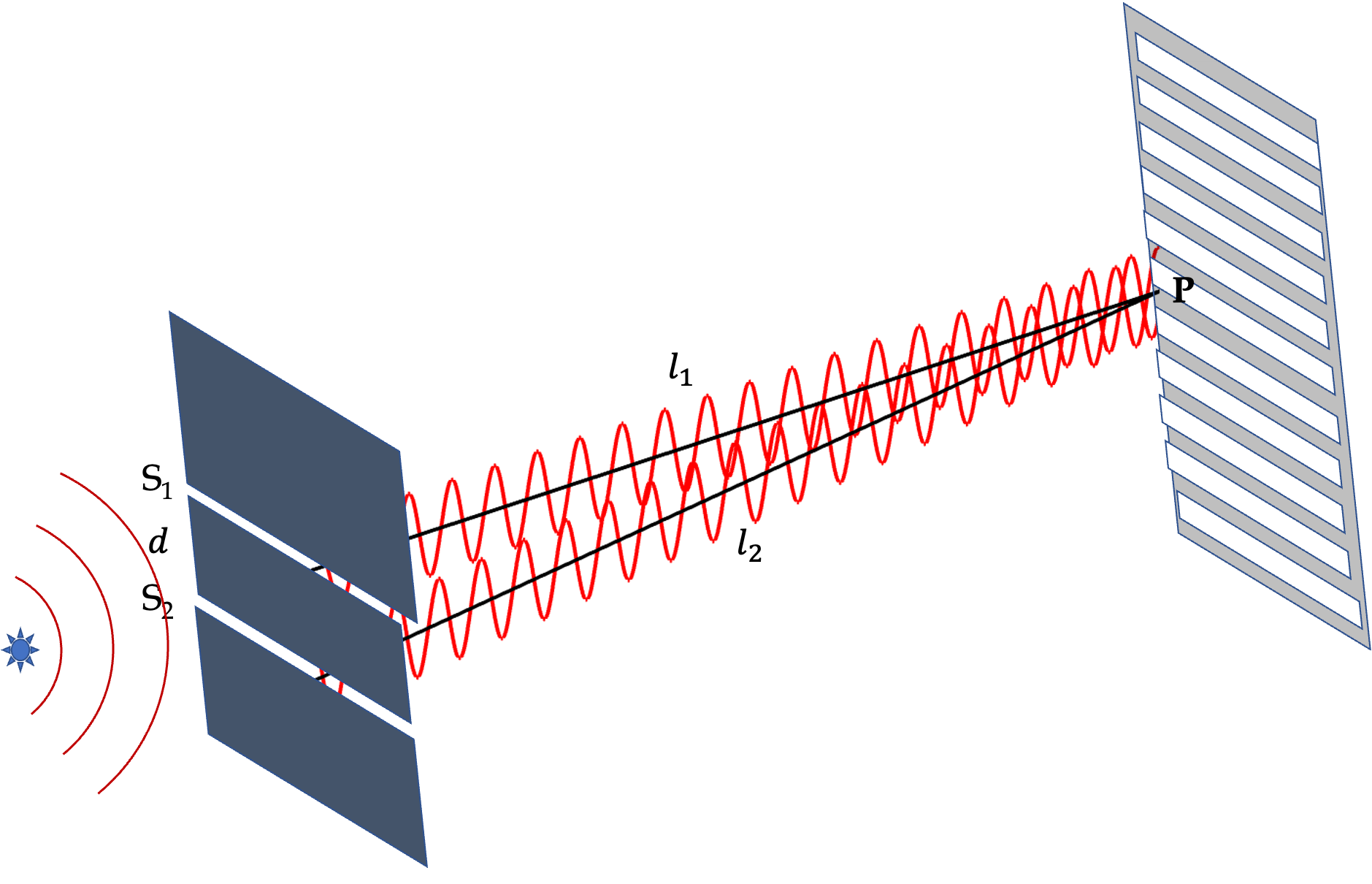Figure 50.2.1. Young's double-slit experimental setup. The two waves from slits $\text{S}_1$ and $\text{S}_2$ start out in-phase at the two slits since they are part of the same wavefront. They travel different distances and interfere on the screen. Their interference produces alternating bright and dark finges on the screen.

We wish to find points on the screen where the interference will be constructive and points where it will be destructive. We will find it most useful to write these conditions in terms of the phase difference between the two waves when they arrive at P since they start out with zero difference.

If the two waves arrive at P completely in sync, i.e., if the difference of their phases is zero or integer multiples of $2\pi\text{,}$ then they will interfere maximally constructive there. On the other hand, if they have opposite phases, meaning the difference in phases are odd multiples of $\pi\text{,}$ then they will maximally destruct.

Let $\Delta_{12}$ be the phase difference between the phases of the two waves arriving there. Then, we can write the following for the conditions for maximal constructive and desctructive interferences.

\begin{align} \amp \text{Constructive: } \Delta_{12} = m \times 2\pi,\ \ (m\ \text{ integer}.) \label{eq-constructive-interference-condition-phase}\tag{50.2.1}\\ \amp \text{Destructive: } \Delta_{12} = m^\prime \times \pi,\ \ (m^\prime\ \text{ odd integer}.) \label{eq-destructive-interference-condition-phase}\tag{50.2.2} \end{align}

In the following, I will show that the phase difference can be expressed in terms of the wavelength $\lambda$ and location of P with respect to the slits in terms of geometric parameters shown in Figure 50.2.3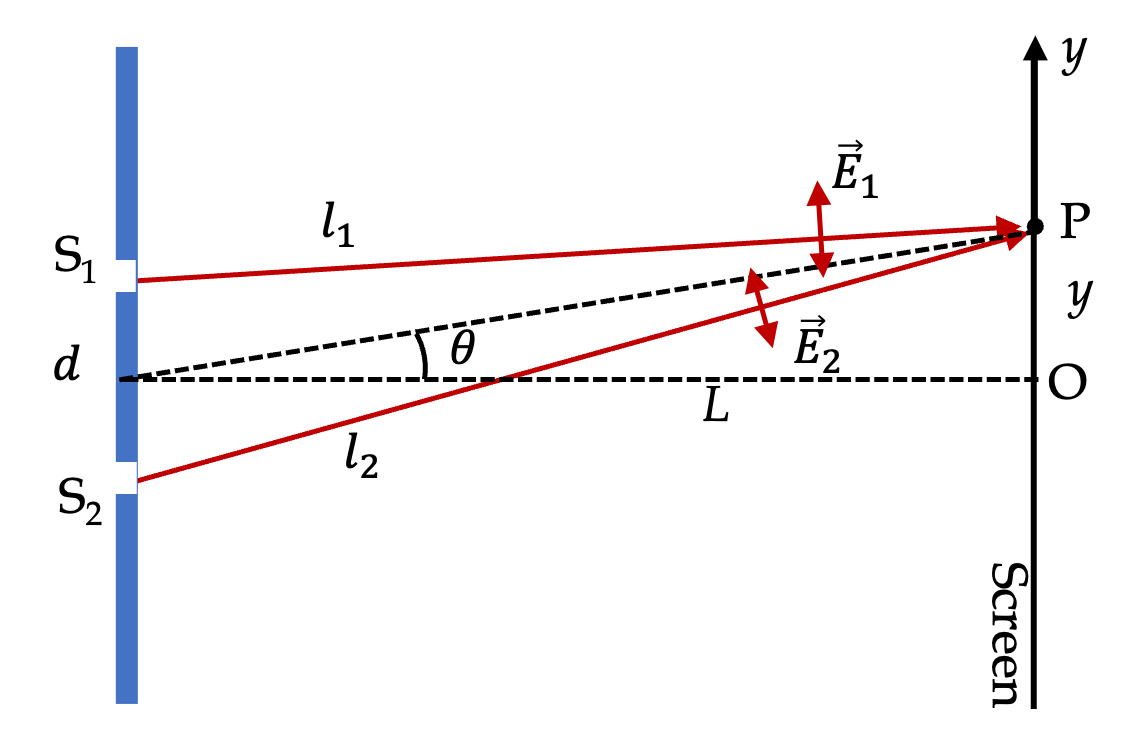Figure 50.2.3. Geometric parameters for Young's double-slit experiment. Also shown are the directions of electric field in the two EM waves.

Let $l_1$ and $l_2$ be the distances $\text{S}_1\text{P}$ and $\text{S}_2\text{P}$ respectively, $d$ the distances between the slits, $\text{S}_1\text{S}_2\text{,}$ and $\theta$ angle from the midpoint between the slits and P. Then, we show in a section below that

\begin{equation} \Delta_{12} = \frac{2\pi}{\lambda} \left( l_1 - l_2\right) \approx \frac{2\pi}{\lambda} \: d\sin\theta,\tag{50.2.3} \end{equation}

where the last step is approximation for small angles, i.e., for P close to the center of the screen.

Using these expressions of the phase difference, we can write the constructive and destructive condition in other forms.

\begin{align} \amp \text{Constructive: } [l_1 - l_2 \text{ or } d\sin\theta] = m \times \lambda,\ \ (m\ \text{ integer}.) \label{eq-constructive-interference-condition-distance}\tag{50.2.4}\\ \amp \text{Destructive: } [l_1 - l_2 \text{ or } d\sin\theta] = m^\prime \times \frac{\lambda}{2},\ \ (m^\prime\ \text{ odd integer}.) \label{eq-destructive-interference-condition-phase-distance}\tag{50.2.5} \end{align}

These forms of the conditions help us relate the interference pattern to distances and hence are extremely useful for applications.

### Subsection50.2.1Intensity on the Screen

Here we will like to find an expression of intensity at an arbitrary point on the screen. We will make simplifying assumptions to make the calculations simpler to work out. Refer to Figure 50.2.3 for symbols we will use. First notice that vector from $\text{S}_1$ to P is in different direction than vector from $\text{S}_2$ to P. Since fields of EM wave are perpendicular to their propagation direction, the electric fields in the two waves will, in general, be oriented diffferently. However, if we are looking at points near the center of the screen, we may ignore their difference. Therefore, we will say that both electric waves have only $y$ component nonzero.

\begin{equation*} \vec E_1 \approx \hat u_y E_{1y},\ \ \ \vec E_2 \approx \hat u_y E_{2y}, \end{equation*}

where $\hat u_y$ is a unit vector in positive $y$ axis. Sometimes this unit vector is denoted by $\hat j\text{.}$ We suppose that amplitude of the two waves are equal and use $E_0$ for that. The wavelength $\lambda$ and hence wavenumber $k$ is same for both and so is the frequency $f$ and angular frequency $\omega\text{.}$ Then, at P, the two waves will have the following expressions.

\begin{align} \amp E_{1y}(\text{at P}) = E_0 \cos(k l_1 - \omega t), \label{eq-wavefunction-wave-from-s1}\tag{50.2.6}\\ \amp E_{2y}(\text{at P}) = E_0 \cos(k l_2 - \omega t), \label{eq-wavefunction-wave-from-s2}\tag{50.2.7} \end{align}

The phase difference, denoted by $\Delta_{12}\text{,}$ will be

\begin{equation} \Delta_{12} = (k l_1 - \omega t) - (k l_2 - \omega t) = k(l_1 - l_2) = \frac{2\pi}{\lambda} (l_1 - l_2). \label{eq-phase-difference-waves-from-s1-and-s2}\tag{50.2.8} \end{equation}

By superposition of waves in Eq. (50.2.6) and (50.2.7), we get $y$ component of the net electric field at P to be

\begin{align*} E_y(\text{at P}) \amp = E_{1y}(\text{at P})+ E_{2y}(\text{at P})\\ \amp = E_0 \cos(k l_1 - \omega t) + E_0 \cos(k l_2 - \omega t). \end{align*}

Intensity will come from time-averaging the square of this field. Let me first write the answer and then give you the calculations.

\begin{equation} I = c\epsilon_0 E_0^2 \left[ 1 + \cos\left( k(l_1-l_2) \right) \right].\label{eq-intensity-at-screen-derived}\tag{50.2.9} \end{equation}

Here is the calculation. Lets use $\ll \cdots \gg$ for time averaging of whatever is enclosed, just not to miss this important thing you must do if you are calculating intensity.

\begin{align*} I(\text{at P}) \amp = \epsilon_0 c \ll E_y^2 (\text{at P}) \gg \\ \amp = \epsilon_0 c E_0^2 \ll \left[ \cos(k l_1 - \omega t) + \cos(k l_2 - \omega t) \right]^2 \gg \\ \amp = \epsilon_0 c E_0^2 \left[ \right.\\ \amp \ \ \ \ \ \ \ \ \ \ \ \ \ll \cos^2 (k l_1 - \omega t)\gg\\ \amp \ \ \ \ \ \ \ \ \ \ + \ll \cos^2 (k l_2 - \omega t)\gg \\ \amp \ \ \ \ \ \ \ \ \ \ \left. + 2 \ll \cos(k l_1 - \omega t) \cos(k l_2 - \omega t) \gg \right] \\ \amp = \epsilon_0 c E_0^2 \left[ \frac{1}{2} + \frac{1}{2} + \cos(kl_1 - kl_2) \right] \\ \amp = \epsilon_0 c E_0^2 \left[ 1 + \cos(k(l_1 - l_2)) \right] \end{align*}

We can write intensity at screen in Eq. (50.2.9) in terms of inensity of each beam. Intensity in beam is

\begin{equation*} I_1 = \frac{1}{2} c \epsilon_0 E_0^2 = I_2. \end{equation*}

Let us denote this by a common symbol $I_0\text{.}$ Now, we write Eq. (50.2.9) as follows.

\begin{equation} I = 2I_0 \left[ 1 + \cos\left( k(l_1-l_2) \right) \right].\tag{50.2.10} \end{equation}

We can write this also in terms of the phase difference between the two waves, which was given in Eq. (50.2.8).

\begin{equation} I = 2I_0 \left[ 1 + \cos\left( \Delta_{12}\right) \right].\label{eq-intensity-at-screen-derived-in-phase-diff}\tag{50.2.11} \end{equation}

This result tells us that intensity will vary between zero and $4I_0\text{,}$ four times the intensity from each slit.

Plotting the net intensity $I$ at point P vs the phase difference $\Delta_{12}$ between two waves when they reach that point shows an interference pattern of maximum and minimum brightness as shown in Figure 50.2.4. When At the center of the screen, the phase difference will be 0 and there will be maximum brightness there. Maximum brightness wil loccur at $\Delta_{12}$ at $\pm 2\pi\text{,}$ $\pm 4\pi\text{,}$ $\pm 6\pi\text{,}$ etc. At these points, the interference term adds to the other terms and constructive interference results. When the phase difference is $\pm \pi\text{,}$ $\pm 3\pi\text{,}$ $\pm 5\pi\text{,}$ etc, the interference term subtracts from the other terms, and a destructive interference takes place. You will see a dark or less bright spot at these locations.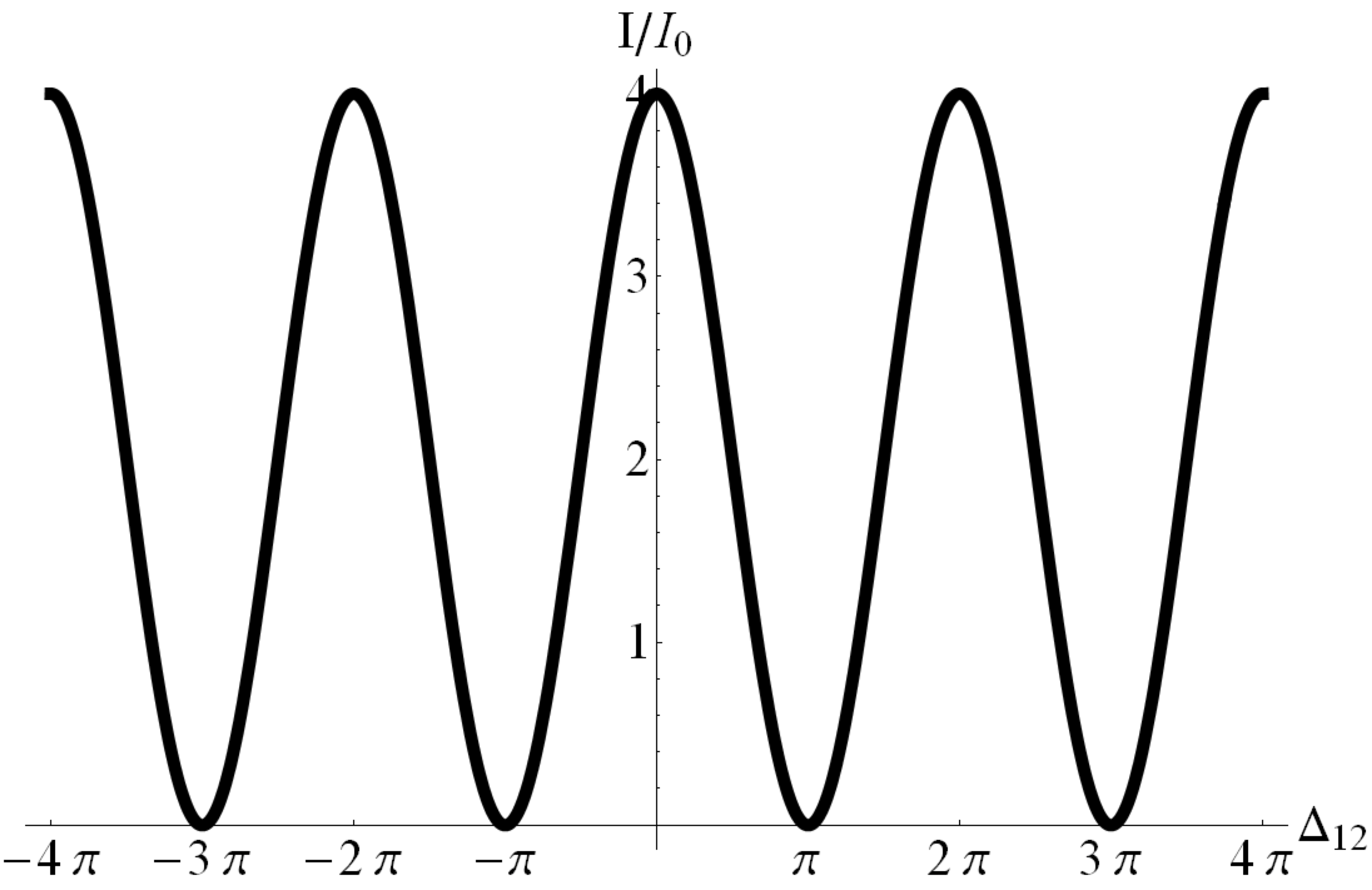Figure 50.2.4. Intensity plotted against the phase difference $\Delta_{12}\text{.}$ The maximally bright spots have $4$ times the intensity of light from each slit while dark spots have zero intensity.

#### Subsubsection50.2.1.1Conditions for Constructive and Destrucive Interferences

From Eq. (50.2.11) we immediately see that intensity will vary depending on the value of $\cos\Delta_{12}\text{.}$ Since cosine has values between $-1$ and $+1\text{,}$ inclusive, the maximum intensity will occur when cosine is $+1$ and minimum when $-1\text{.}$ Therefore,

\begin{align*} \amp \text{Constructive when }\cos\Delta_{12} = + 1. \\ \amp \text{Desructve when }\cos\Delta_{12} = -1. \end{align*}

This will happen at the following values of the phase difference

\begin{align*} \amp \text{Constructive when }\Delta_{12} = 0, \pm 2\pi, \pm 4\pi, \cdots. \\ \amp \text{Desructve when }\Delta_{12} =0, \pm \pi, \pm 3\pi, \cdots. \end{align*}

Of course, we can write this in another way

\begin{align} \amp \text{Constructive when }\Delta_{12} = m \times 2\pi \ \ (m \text{ an integer}). \tag{50.2.12}\\ \amp \text{Desructve when }\Delta_{12} =m^\prime \times \pi \ \ (m^\prime \text{ an odd integer}). \tag{50.2.13} \end{align}

Using the expression of phase difference between two waves given in Eq. (50.2.8) we get yet another form of the extreme interference conditions. Write $k$ as $2\pi/\lambda\text{,}$ we get

\begin{align} \amp \text{Constructive when } l_1-l_2 = m \lambda \ \ (m \text{ an integer}). \label{eq-constructive-interference-conditions-youngs-in-lengths}\tag{50.2.14}\\ \amp \text{Desructve when } l_1-l_2 =m^\prime \frac{\lambda}{2}\ \ (m^\prime \text{ an odd integer}). \label{eq-destructive-interference-conditions-youngs-in-lengths}\tag{50.2.15} \end{align}

This makes sense when you look at what happens to the waves. They start out at the slits in-step with each other, and when they travel to different distances, they will tend to go out of step by varying amounts. They will be completely in-step at places where path difference is an integer multiple of one wavelength, and they will be completely out of step, meaning if one is at the crest, then the other would be at the trough there, if the difference in path is off by an odd multiple of half a wavelength.

The values of $m$ and $m^\prime$ are called order of interference or interference order. For example, when we are looking at $m=2\text{,}$ we say it is second order constructive interference, and when looking at $m^\prime\text{,}$ we say it is second order destructive inerference.

#### Subsubsection50.2.1.2Conditions Near Center of Screen

Equations (50.2.14) and (50.2.15) are conditions for maximum and minimum intensity at a point on the screen in terms of the distances to the point from the two slits. Most useful applications involve patterns near the center or the symmetric point on the screen. In these cases, we speak of the direction from slits to the point in which we will have maxima and minima of intensity.

Figure 50.2.5 shows a way to write $|l_1-l_2|$ in terms of angle $\theta\text{.}$ Suppose we are looking at points above the symmetric divider between the slits as in the figure. This will mean $l_1\lt l_2$ for this illustration. We will then draw an arc with center at P and radius equal to $l_1\text{,}$ which will intersect the $l_2$ line at A. That means $\text{S}_2\text{A}$ will be equal to $l_2-l_1\text{.}$ Now, this arc is very small part of a large circle. So, if we draw a triangle with points $\text{S}_1\text{,}$ $\text{S}_2\text{,}$ and A, this triangle is almost a right angled triangle with $\angle A \text{S}_1 \text{S}_2 = \theta$ and $\angle \text{S}_1 \text{A} \text{S}_2 = 90^\circ\text{.}$ Using this triangle we get the following difference in paths in terms of the distance $d$ between the slits and direction angle $\theta$ from the slits.

\begin{equation*} |l_1 - l_2| = d\sin\theta. \end{equation*}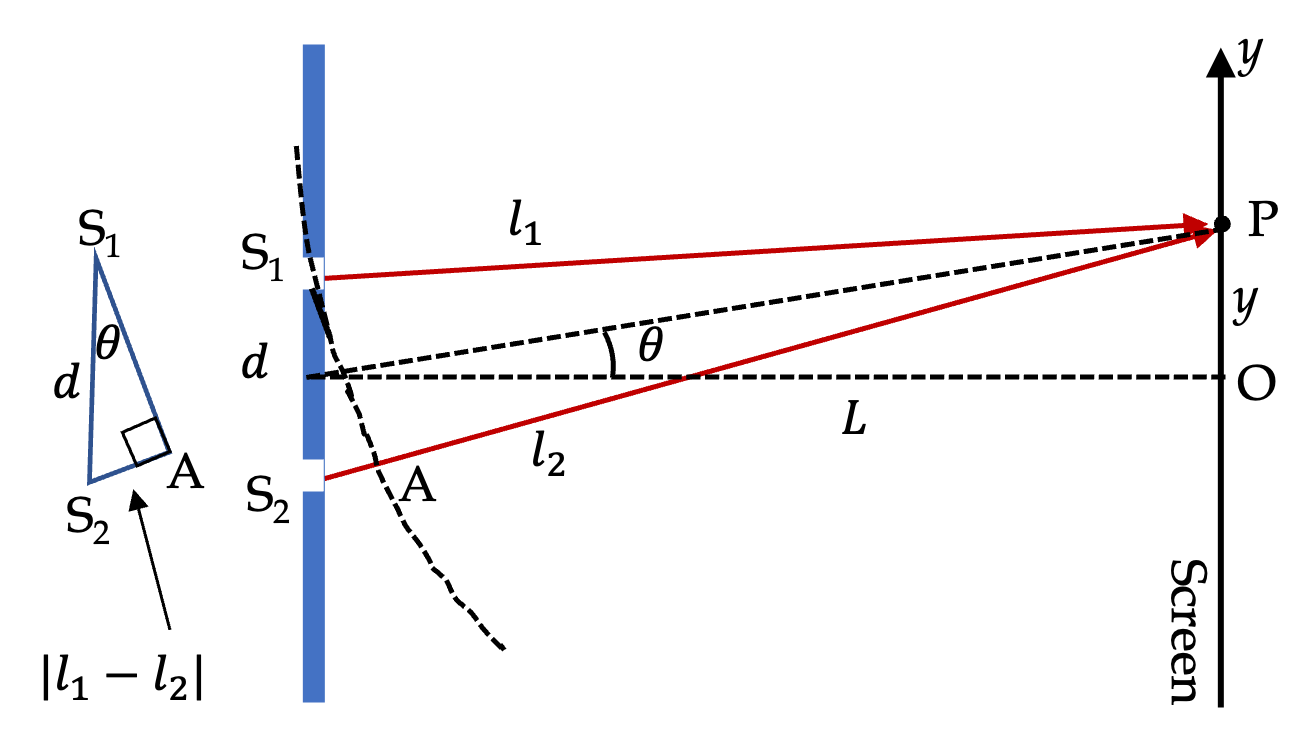Figure 50.2.5. Geometric parameters for Young's double-slit experiment near center of the screen where angle $\theta$ from center of the slits to a point on te the screen is small.

We can take the absolute value out if we think of $\theta$ positive for points above the center line and negative for points below the center line.

\begin{equation*} l_2 - l_1 = d\sin\theta. \end{equation*}

Replacing $l_1-l_2$ in the interference conditions, we get the following useful form of the interference conditions.

\begin{align} \amp \text{Constructive when } d\sin\theta = m \lambda \ \ (m \text{ an integer}). \label{eq-constructive-interference-conditions-youngs-in-d-theta}\tag{50.2.16}\\ \amp \text{Desructve when } d\sin\theta =m^\prime \frac{\lambda}{2}\ \ (m^\prime \text{ an odd integer}). \label{eq-destructive-interference-conditions-youngs-in-d-theta}\tag{50.2.17} \end{align}

These give one value of $\theta$ for each $m$ and $m^\prime\text{.}$ Often we attach subscript to $\theta$ with the value of $m$ and $m^\prime\text{,}$ as in $\theta_1\text{,}$ $\theta_2\text{,}$ etc., meaning

\begin{equation*} d\sin\theta_1 = 1\times \lambda,\ \ \ d\sin\theta_2 = 2\times \lambda. \end{equation*}

We can equivalently write these conditions in terms of location of point P on the screen. Figure 50.2.5 shows a $y$ axis on the screen that is vertically aligned. We note that for points near the center at a far away screen, i.e., when angle is small, or equivalently, when $y \ll L$ we will have

\begin{equation*} \sin\theta = \frac{y}{\sqrt{y^2 + L^2}} \approx \frac{y}{L}. \end{equation*}

Therefore, the interference conditions in terms of position on the screen will be

\begin{align} \amp \text{Constructive when } y = m \frac{\lambda L}{d} \ \ (m \text{ an integer}). \label{eq-constructive-interference-conditions-youngs-in-y}\tag{50.2.18}\\ \amp \text{Desructve when } d\sin\theta =m^\prime \frac{\lambda L}{2d} \ \ (m^\prime \text{ an odd integer}). \label{eq-destructive-interference-conditions-youngs-in-y}\tag{50.2.19} \end{align}

Figure 50.2.6 shows a simulation of two slits separated by 4-wavelengths. One can see the bright and dark fringes emanating in different directions from the sources. Looking at the screen on the right, you see bright and dark fringes.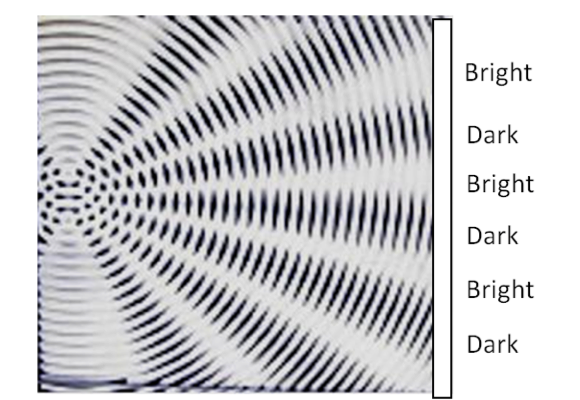Figure 50.2.6. Demosntration of interference pattern in Young's double-slit experiment. Two plastic plates with bright and dark circles displaced a small distance, simulating two sources, gives the pattern of constructive and destructive patterns in different directions.

#### Subsubsection50.2.1.3Maximum Number of Fringes

Notice that in the condition for constructive interference given in Eq. (50.2.16), after you divide both sides by $D\text{,}$ you can will get the condition as the sine of angle.

\begin{equation*} \text{Constructive when } \sin\theta = m \frac{\lambda}{d} \ \ (m \text{ an integer}). \end{equation*}

The order number $m$ is supposed to be an integer, but it cannot just grow to any value since since function is limited to be within $-1$ and $+1\text{.}$ Demanding this we get maximum value for $m$ that will be allowed. Let's denote it by $m_\text{max}\text{.}$

\begin{equation*} 1 = m_\text{max} \frac{\lambda}{d}. \end{equation*}

Solving this for floor of the integer we get the following for the highest value of positive $m$ allowed.

\begin{equation} m_\text{max} = \left\lfloor\frac{d}{\lambda} \right\rfloor.\label{eq-max-constructive-m-allowed}\tag{50.2.20} \end{equation}

Similarly, maximum positive $m^\prime$ value is limited by the destructive interference condition.

\begin{equation} m^\prime_\text{max} = \left\lfloor\frac{2d}{\lambda} \right\rfloor.\label{eq-max-destructive-m-prime-allowed}\tag{50.2.21} \end{equation}

Thus, if $d = 0.6\lambda\text{,}$ then $m_\text{max}=0$ and $m^\prime_\text{max}=1\text{.}$ That means you will see one bright fringe surrounded by the $m^\prime=\pm 1$ dark fringes, and then some brigtness will surround them, but you will not get $m=\pm 1$ bright fringes. If $d = 2.8\lambda\text{,}$ then $m_\text{max}=2$ and you will see only five bright fringes, namely, $m=-2, -1, 0, 1, 2\text{,}$ each surrounded by dark fringes.

Note that Eqs. (50.2.20) and (50.2.21) may give inconsistent values. You will need to make sure that each constructive fringe is bounded by a destructive fringe and vice versa. For instance, in Checkpoint 50.2.7, you will notice that $m_\text{max}=2$ and $m^\prime_\text{max}=4\text{.}$ Clearly, between $m^\prime=3$ and $m^\prime=4\text{,}$ there is no bright fringe in this case. Here, we will just stop at $m=2$ and $m^\prime=3$ on the positive order side and $m=-2$ and $m^\prime=-3$ at the negative order side.

A red light of wave length $0.63\,\mu\text{m}$ is incident on a double-slit system with slits separated by $1.50\,\mu\text{m}\text{.}$ A screen is place at a distance of $0.5\text{ m}$ from the slits. Suppose the slits are along $y$axis with origin at the midpoint of their separation. Find approximate $y$ coordinates of locations on the screen where (a) intensity is maximum and (b) intensity is minimum. You can use small angle aproximation since only approximate values of $y$ are required.

Hint

First find maximum number of fringes you will see and then use conditions given in $y\text{.}$

(a) $0,\ \pm 21\text{ cm},\ \pm 42\text{ cm}\text{,}$ (b) $\pm 10.5\text{ cm},\ \pm 31.5\text{ cm}\text{.}$

Solution

(a) and (b) Let's first find the maximum number of bright and dark fringes we will see. We have $d = 1.5\ \mu\text{m}$ and $\lambda = 0.63\ \mu\text{m}\text{.}$ Hence the maximum positive order of constructive and destructive fringes will be

\begin{align*} m_{\text{max}} \amp= \left \lfloor \frac{1.5\ \mu\text{m}}{0.63\ \mu\text{m}} \right\rfloor = 2.\\ m^\prime_{\text{max}} \amp= \left \lfloor \frac{2\times 1.5\ \mu\text{m}}{0.63\ \mu\text{m}} \right\rfloor = 4. \end{align*}

Since around the bright fringe order $m=2$ we will have $m^\prime=2$ and $m^\prime=3$ dark fringes and there is no more bright fringe. Therefore, theoretically, we will have $m=0, \pm 1, \pm 2$ for the bright fringes and $m^\prime = \pm 1, \pm 2, \pm 3$ for the dark fringes. We will further limit our calculation to $|m^\prime|=2$ since by the time we get to $m=2$ fringe, angles are already getting too large and the formula becomes quite inaccurate.

Since we will encounter $\lambda L/d\text{,}$ we calculate it once.

\begin{equation*} \frac{\lambda L}{d} = \frac{ 0.63\ \mu\text{m}\times 0.5\text{ m} }{1.50\ \mu\text{m}} = 21\text{ cm}. \end{equation*}

(a) The $y$ coordinates of the constructive interference maxima are

\begin{align*} \amp m = 0:\ \ y=0 \\ \amp m = \pm 1:\ \ y= \pm 21\text{ cm}\\ \amp m = \pm 2:\ \ y= \pm 42\text{ cm} \end{align*}

(b) The $y$ coordinates of the destructive interference are

\begin{align*} \amp m^\prime = \pm 1:\ \ y= \pm 10.5\text{ cm}\\ \amp m = \pm 2:\ \ y= \pm 31.5\text{ cm} \end{align*}

A laser light of wavelength $632.8\text{ nm}$ passes through two narrow slits separated by $10.0\ \mu\text{m}$ and form an interference pattern on a screen $1.5\text{ m}$ away. (a) Find the location of three bright and two dark spots. (b) What is the maximum order of interference seen on the screen?

Hint

Use interference conditions.

(a) Bright: $0^\circ\text{,}$ $+3.63^\circ\text{,}$ $-3.63^\circ\text{;}$ Dark: $+1.81^\circ\text{,}$ $-1.81^\circ$ (b) $15 \text{.}$

Solution 1 (a)

(a) First let us find the positions of the constructive interferences. Here they occur in the directions given by the angle $\theta$ that obeys

\begin{equation*} d\sin\theta = m\lambda,\ \ m = 0, \pm 1, \pm 2, \cdots. \end{equation*}

This gives the following angles for three values of $m\text{.}$

\begin{align*} \amp m = 0 \ \ \ \Longrightarrow\ \ \ \theta = 0.\\ \amp m = 1 \ \ \ \Longrightarrow\ \ \ \theta = \sin^{-1}\left(\dfrac{\lambda}{d} \right) = +3.63^{\circ}.\\ \amp m = -1 \ \ \ \Longrightarrow\ \ \ \theta = \sin^{-1}\left(\dfrac{-\lambda}{d} \right) = -3.63^{\circ}. \end{align*}

Here, the destructive interferences occur at the angle given by

\begin{equation*} d\sin\theta = \left(m + \dfrac{1}{2}\right)\:\lambda,\ \ m = 0, \pm 1, \pm 2, \cdots. \end{equation*}

We will find the angles for $m = 0$ and $m = -1\text{.}$

\begin{align*} \amp m = 0 \ \ \ \Longrightarrow\ \ \ \theta = \sin^{-1}\left(\dfrac{\lambda}{2d} \right) = +1.81^{\circ}.\\ \amp m = -1 \ \ \ \Longrightarrow\ \ \ \theta = \sin^{-1}\left(\dfrac{-\lambda}{2d} \right) = -1.81^{\circ}. \end{align*}
Solution 2 (b)

(b) $n_{\text{max}} = \left\lfloor\frac{d}{\lambda} \right\rfloor = 15\text{.}$

The sunlight is incident on an opaque board with two narrow slits separated by 250 $\mu$m. A spectrum of bands is observed on a white board screen one meter away from the slits. (a) What is the separation between the red (assume 650 nm) and blue (assume 400 nm) bands in the first order? (b) What is the separation between the red (assume 650 nm) and blue (assume 400 nm) bands in the second order?

Hint

Use interference conditions.

(a) $y_{\text{red}} = 2.6\ \text{mm}\text{,}$ $y_{\text{blue}} = 1.6\ \text{mm}\text{;}$ (b) $y_{\text{red}} = 5.2\ \text{mm}\text{,}$ $y_{\text{blue}} = 3.1\ \text{mm}\text{.}$

Solution 1 (a)

(a) (Red) We will first find the angles and positions on the screen for the first and second order interferences and then use them to answer the two parts.

\begin{align*} \amp \theta_1 (\text{red})= \sin^{-1}\left(\dfrac{650\:\text{nm}}{250\times 10^{3}\:\text{nm}} \right) = 0.149^{\circ},\\ \amp \theta_2 (\text{red})= \sin^{-1}\left(\dfrac{2\times650\:\text{nm}}{250\times 10^{3}\:\text{nm}} \right) = 0.298^{\circ}. \end{align*}

We convert these to $y$ coordinates at the screen by $\tan\theta = y/L\text{.}$

\begin{align*} \amp y_1(\text{red}) = 1000\text{ mm}\tan 0.149^{\circ} = 2.6\:\text{mm},\\ \amp y_2(\text{red}) = 1000\text{ mm}\tan 0.298^{\circ} = 5.2\:\text{mm} \end{align*}
Solution 2 (b)

(b) (Blue)

\begin{align*} \amp \theta_1 (\text{blue})= \sin^{-1}\left(\dfrac{4000\:\text{nm}}{250\times 10^{3}\:\text{nm}} \right) = 0.092^{\circ},\\ \amp \theta_2 (\text{blue})= \sin^{-1}\left(\dfrac{2\times400\:\text{nm}}{250\times 10^{3}\:\text{nm}} \right) = 0.18^{\circ}. \end{align*}

We convert these to $y$ coordinates at the screen by $\tan\theta = y/L\text{.}$

\begin{align*} \amp y_1(\text{blue}) = 1000\text{ mm}\tan 0.092^{\circ} = 1.6\:\text{mm},\\ \amp y_2(\text{blue}) = 1000\text{ mm}\tan 0.18^{\circ} = 3.2\:\text{mm}. \end{align*}

When a laser light of unknown wavelength is passed through two narrow slits separated by 150 $\mu$m, an interference pattern is observed on a screen 1.5 m away. The distance between the central spot and first order constructive interference is found to be 5 mm. (a) Find the wavelength of light. (b) Where would the second order constructive interference form on the screen, if at all?

Hint

Using small angle, you should get $\lambda \approx d \times \dfrac{y}{L}\text{.}$

(a) $0.5\:\mu\text{m}\text{.}$ (b) $10\:\text{mm}\text{.}$

Solution 1 (a)

(a) Let $\lambda$ be the unknown wavelength and let $d$ stand for the separation of the slits. Then the condition for the angle $\theta$ for a first-order constructive interference in this situation will be

\begin{equation*} d\:\sin\:\theta = \lambda. \end{equation*}

The angle $\theta$ is also obtained from the geometry of set up. From the distance $L$ to the screen and the distance $y$ of the interference spot from the central spot we get

\begin{equation*} \sin\theta = \dfrac{y}{\sqrt{y^2 + L^2}} \approx \dfrac{y}{L}. \end{equation*}

Therefore,

\begin{equation*} \lambda = d \times \dfrac{y}{L}. \end{equation*}

Now, we can put in the numerical values to obtain

\begin{equation*} \lambda = 150\:\mu\text{m}\times \dfrac{0.005\:\text{m}}{1.5\:\text{m}} = 0.5\:\mu\text{m}. \end{equation*}
Solution 2 (b)

(b) For the second order interference the condition is

\begin{equation*} d\:\sin\:\theta = 2\lambda,\ \ \sin\:\theta = \dfrac{2\lambda}{d} = \dfrac{1\:\mu\text{m}}{150\:\mu\text{m}}. \end{equation*}

Therefore, $\theta \approx 1/150\:\text{rad}\text{.}$ Now, we multiply this by $L= 1.5$ m to obtain the $y$-coordinate of this spot, $y = \frac{1}{150}\:\text{rad} \times 1500\:\text{mm} = 10\:\text{mm}\text{.}$